# Predicting car prices with machine learning

How to use data science to buy a car

The process of buying a pre-owned car can take a lot of time. You can find thousands of cars online and offline and you can easily spend hours or days comparing them as the pricing of pre-owned cars is not very transparent. Therefore, I decided to search for a car in the nerdiest way possible: by scraping a car website and creating a model to predict the market value of pre-owned cars.

# The data

Since I want to have a dataset to both train the machine learning model and to select potential cars to buy, I decided to scrape a Dutch car website myself instead of using an old Kaggle dataset. The scraping can be done with Selenium or BeautifulSoup and this resulted in a dataset of c. 45k pre-owned cars with prices between €5k and €25k, excluding diesel cars.

After cleansing the data (and checking the distribution), the dataset includes many relevant features that can be used to predict the market value of each car in the dataset.

Checking the distribution of cars by manufacturer shows that the number 1 manufacturer in my dataset is Volkswagen, followed by Opel and Renault and the majority of cars in my dataset are hatchbacks followed by SUVs.

`plt.figure(figsize = (20,15))cars['Brand'].value_counts().plot(kind='bar')`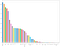Distribution of cars by brand
`plt.figure(figsize = (20,15))cars['Type'].value_counts().plot(kind='bar')`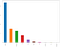Distribution of cars by type

To visualize the dataset and to better understand the relationships between the variables, we can create a correlation heatmap. This heatmap shows high positive correlations between the variables year and price, and between the new price of the car and the actual price. A high negative correlation can be observed between the variables year and km (odometer). These correlations make sense, so this is a good starting point for the data modeling part.

`# calculate correlation matrixcorr = cars.corr()# plot the heatmapplt.figure(figsize =(20,15))ax=sns.heatmap(corr, xticklabels=corr.columns, yticklabels=corr.columns, annot=True, cmap=sns.diverging_palette(220, 20, as_cmap=True))bottom, top = ax.get_ylim()ax.set_ylim(bottom + 0.5, top - 0.5)`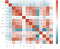Correlation heatmap

# Data modeling

To predict the market values of the cars in my dataset, I use a random forest model. Before I can start modeling I first have to transform the categorical variables into dummy variables to transform every unique value of a variable into its own binary variable.

`cars = pd.get_dummies(cars, drop_first=True)`

Secondly, I need to scale the independent variables. The scaling of the independent variables is important because the max value of the year variable is 2021, whereas the max number for km (odometer) is 385,000 km. Therefore, if you wouldn’t scale the data a small change in the km variable would have a much larger impact on the model compared to the same change in the year variable.

`from sklearn.preprocessing import StandardScalerX_head = cars.iloc[:, cars.columns != 'Price']X = cars.loc[:, cars.columns != 'Price']y = cars['Price']X = StandardScaler().fit_transform(X)`

Now we can train and test the random forest model. The random forest basically builds multiple decision trees and merges them together (it operates as an ensemble) to get a more accurate and stable prediction. I use 80% of the dataset to train the model and 20% for testing it.

`from sklearn.ensemble import RandomForestRegressorfrom sklearn.model_selection import train_test_splitfrom sklearn.metrics import mean_absolute_error as maeX_train, X_test, y_train, y_test = train_test_split(X, y, test_size=.20, random_state=0)model = RandomForestRegressor(random_state=1, max_depth=50)model.fit(X_train, y_train)pred = model.predict(X_test)print(mae(y_test, pred))print(cars['Price'].mean())model.score(X_test,y_test)`

The model resulted in an MAE (mean absolute error) of 896 for a mean price of €13,498 and achieved an accuracy score of 94.3%!

We can visualize one single decision tree of the random forest model with the following code:

`from sklearn.tree import export_graphvizfrom sklearn import treefrom sklearn.datasets import load_bostonfrom dtreeviz.trees import dtreeviz # will be used for tree visualizationfrom matplotlib import pyplot as pltplt.rcParams.update({'figure.figsize': (12.0, 8.0)})plt.rcParams.update({'font.size': 14})plt.figure(figsize=(20,20))treepicture = tree.plot_tree(model.estimators_, feature_names=cars.columns, filled=True)`

It is impossible to read the entire decision tree, so we can limit the depth of the decision tree to check a single decision tree with a limited number of variables. To classify a new point, simply move down the tree, using the features of the point to answer the questions until you arrive at a leaf node where the class is the prediction. But keep in mind that all variables are scaled, so all the feature values in the tree are also scaled.

Checking the feature importance shows that the year variable and new_price variable are the key features to calculate the market value of used cars, followed by the KM (odometer) variable.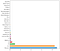Feature importance of the model

Since the new_price of the car also indirectly accounts for other car features, I also train the model without this variable. This would still result in an accuracy score of 93.8%. The car features, such as horsepower (PK variable), have become more important in this model.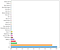Feature importance of the model without the new price variable

I used the trained model to create a dataset of thousands of cars including car features, the price, and the estimated market value based on the random forest model. This allowed me to filter out the best car deals and explore these cars in further detail. The model also allowed me to check the estimated market value of cars that I spotted on other websites and offline, and a couple of days ago I bought a car based on this small data science project!

Thank you for reading! If you have any questions or comments regarding this article, please feel free to comment below.

## MLearning.ai

Data Scientists must think like an artist when finding a solution

## MLearning.ai

Data Scientists must think like an artist when finding a solution, when creating a piece of code.Artists enjoy working on interesting problems, even if there is no obvious answer.

Written by

## Melissa de Beyer

Senior consultant and self-taught data scientist| Linkedin: https://www.linkedin.com/in/melissadebeyer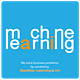## MLearning.ai

Data Scientists must think like an artist when finding a solution, when creating a piece of code.Artists enjoy working on interesting problems, even if there is no obvious answer.

## More From Medium

Medium is an open platform where 170 million readers come to find insightful and dynamic thinking. Here, expert and undiscovered voices alike dive into the heart of any topic and bring new ideas to the surface. Learn more

Follow the writers, publications, and topics that matter to you, and you’ll see them on your homepage and in your inbox. Explore

If you have a story to tell, knowledge to share, or a perspective to offer — welcome home. It’s easy and free to post your thinking on any topic. Write on Medium